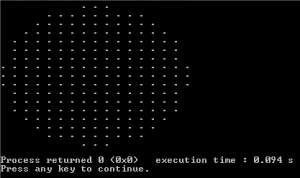Related Articles
Draw a circle without floating point arithmetic
• Difficulty Level : Hard
• Last Updated : 18 Apr, 2019

Given a radius of a circle, draw the circle without using floating point arithmetic.

Following program uses a simple concept. Let the radius of the circle be r. Consider a square of size (2r+1)*(2r+1) around the circle to be drawn. Now walk through every point inside the square. For every every point (x,y), if (x, y) lies inside the circle (or x^2+ y^2 < r^2), then print it, otherwise print space.

## C++

 `// C++ code to demonstrate to draw``// circle without floating ``// point arithmetic``#include `` ` `void` `drawCircle(``int` `r)``{``    ``// Consider a rectangle of size N*N``    ``int` `N = 2*r+1;`` ` `    ``int` `x, y;  ``// Coordinates inside the rectangle`` ` `    ``// Draw a square of size N*N.``    ``for` `(``int` `i = 0; i < N; i++)``    ``{``        ``for` `(``int` `j = 0; j < N; j++)``        ``{``            ``// Start from the left most corner point``            ``x = i-r;``            ``y = j-r;`` ` `            ``// If this point is inside the circle, print it``            ``if` `(x*x + y*y <= r*r+1 )``                ``printf``(``"."``);``            ``else` `// If outside the circle, print space``                ``printf``(``" "``);``            ``printf``(``" "``);``        ``}``        ``printf``(``"\n"``);``    ``}``}`` ` `// Driver Program to test above function``int`  `main()``{``    ``drawCircle(8);``    ``return` `0;``}`

## Java

 `// Java code to demonstrate to draw``// circle without floating ``// point arithmetic`` ` `class` `GFG``{``static` `void` `drawCircle(``int` `r)``{``    ``// Consider a rectangle of size N*N``    ``int` `N = ``2``*r+``1``;`` ` `    ``int` `x, y; ``// Coordinates inside the rectangle`` ` `    ``// Draw a square of size N*N.``    ``for` `(``int` `i = ``0``; i < N; i++)``    ``{``        ``for` `(``int` `j = ``0``; j < N; j++)``        ``{``            ``// Start from the left most corner point``            ``x = i-r;``            ``y = j-r;`` ` `            ``// If this point is inside the circle, print it``            ``if` `(x*x + y*y <= r*r+``1` `)``                ``System.out.print(``"."``);``            ``else` `                ``// If outside the circle, print space``                ``System.out.print(``" "``);`` ` `            ``System.out.print(``" "``);``        ``}`` ` `        ``System.out.println();``    ``}``}`` ` `// Driver Program to test above function``public` `static` `void` `main(String arg[])``{``    ``drawCircle(``8``);``}``}`` ` `// This code is contributed``// by Anant Agarwal.`

## Python3

 `# Python3 code to demonstrate to draw``# circle without floating``# point arithmetic`` ` `def` `drawCircle(r):`` ` `    ``# Consider a rectangle of size N*N``    ``N ``=` `2` `*` `r ``+` `1`` ` `    ``# Draw a square of size N*N.``    ``for` `i ``in` `range``(N):``        ``for` `j ``in` `range``(N):`` ` `            ``# Start from the left most corner point``            ``x ``=` `i ``-` `r``            ``y ``=` `j ``-` `r`` ` `            ``# If this point is inside the circle, ``            ``# print it``            ``if` `x ``*` `x ``+` `y ``*` `y <``=` `r ``*` `r ``+` `1``:``                ``print``(``"."``, end ``=` `" "``)``                 ` `            ``# If outside the circle, print space``            ``else``:``                ``print``(``" "``, end ``=` `" "``)``        ``print``()`` ` `# Driver Code``if` `__name__ ``=``=` `"__main__"``:``    ``drawCircle(``8``)`` ` `# This code is contributed ``# by vibhu4agarwal`

## C#

 `// C# code to demonstrate to draw ``// circle without floating ``// point arithmetic ``using` `System;`` ` `public` `class` `GFG{``    ``static` `void` `drawCircle(``int` `r) ``{ ``    ``// Consider a rectangle of size N*N ``    ``int` `N = 2*r+1; `` ` `    ``int` `x, y; ``// Coordinates inside the rectangle `` ` `    ``// Draw a square of size N*N. ``    ``for` `(``int` `i = 0; i < N; i++) ``    ``{ ``        ``for` `(``int` `j = 0; j < N; j++) ``        ``{ ``            ``// Start from the left most corner point ``            ``x = i-r; ``            ``y = j-r; `` ` `            ``// If this point is inside the circle, print it ``            ``if` `(x*x + y*y <= r*r+1 ) ``                ``Console.Write(``"."``); ``            ``else``                ``// If outside the circle, print space ``                ``Console.Write(``" "``); `` ` `            ``Console.Write(``" "``); ``        ``} `` ` `        ``Console.WriteLine(); ``    ``} ``} `` ` `// Driver Program to test above function``    ``static` `public` `void` `Main (){``        ``drawCircle(8); ``     ` `      ` `    ``}``// This code is contributed ``// by ajit. ``}`

## PHP

 ``

Output:Please write comments if you find anything incorrect, or you want to share more information about the topic discussed above

Attention reader! Don’t stop learning now. Get hold of all the important DSA concepts with the DSA Self Paced Course at a student-friendly price and become industry ready.

My Personal Notes arrow_drop_up